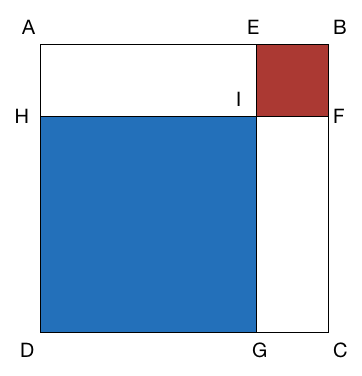# Square display

Geometry Level 2In the above image, $ABCD, EBFI,HIGD$ are all squares.

If the area of $HIGD$ is 16 and the ratio of areas of $HIGD$ to $EBFI$ is $4:1$, what is the area of $ABCD$?

×

Problem Loading...

Note Loading...

Set Loading...